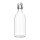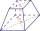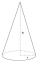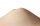# Ice floe

What is the volume of an ice floe weighing 326 kg? The density of ice is only 920 kg/m3.

Correct result:

V =  0.3543 m3

#### Solution:We would be pleased if you find an error in the word problem, spelling mistakes, or inaccuracies and send it to us. Thank you!Tips to related online calculators
Tip: Our volume units converter will help you with the conversion of volume units.
Tip: Our Density units converter will help you with the conversion of density units.

## Next similar math problems:

• AngieAngie poured 4 cartons of milk, each with 2.4 litres, into a mixing bowl. How many litres of milk were there altogether?
• ZhiweiZhiwei wants to divide 19 litres of water equally into 6 pots. Find the volume of water in each pot?
• Wooden bowls20 wooden bowls in the shape of a truncated cone should be painted on the outside and inside with wood varnish. We need 0.1 l of paint to paint 200 cm2. How many liters of paint do we have to buy if the bowls are 25 cm high, the bottom of the bowl has a d
• Fresh juiceThe seller offers fresh-squeezed juice, which the customer either pours into his own containers or sells it in liter plastic bottles, which the customer buys from him. A liter of juice costs 40 CZK more than a bottle. There is no charge for turning the ju
• Pentagonal pyramidFind the volume and surface of a regular pentagonal pyramid with a base edge a = 12.8 cm and a height v = 32.1 cm.
• Cuboid diagonalsThe cuboid has dimensions of 15, 20 and 40 cm. Calculate its volume and surface, the length of the body diagonal and the lengths of all three wall diagonals.
• Cargo spaceThe cargo space of the truck has the dimensions a = 4.2 m, b = 1.9 m, c = 8.1 dm. Calculate its volume.
• Two vasesMichaela has two vases in her collection. The first vase has the shape of a cone with a base diameter d = 20 cm; the second vase has the shape of a truncated cone with the diameter of the lower base d1 = 25 cm and with the diameter of the upper base d2 =
• Triangular pyramidCalculate the volume of a regular triangular pyramid with edge length a = 12cm and pyramid height v = 20cm.
• The woodenThe wooden block measures 12 cm, 24 cm, and 30 cm. Peter wants to cut it into several identical cubes. At least how many cubes can he get?
• From plasticineMichael modeled from plasticine a 15 cm high pyramid with a rectangular base with the sides of the base a = 12 cm and b = 8 cm. From this pyramid, Janka modeled a rotating cone with a base diameter d = 10 cm. How tall was Janka's cone?
• Volume per timeHow long does fill take for a pump with a volume flow of 200 l per minute fill a cube-shaped tank up to 75% of its height if the length of the cube edge is 4 m?
• Sphere cutA sphere segment is cut off from a sphere k with radius r = 1. The volume of the sphere inscribed in this segment is equal to 1/6 of the volume of the segment. What is the distance of the cutting plane from the center of the sphere?
• Into boxHow many cubes with an edge of 2.5 cm fit into a box measuring 11.6 cm; 8.9 cm and 13.75 cm?
• SandHow much m ^ 3 of sand can be loaded on a car with a load capacity of 5 t? The sand density is 1600 kg/m ^ 3 .
• Concrete columnThe concrete column of the highway bridge has the shape of a block with dimensions of 1m x 0.8m x 25m. It should be lifted by crane to a height of 20m. What is the power of his engine if the lifting takes 2 minutes?
• FlowerpotThe block-shaped flowerpot has external dimensions: length 1.25 m, width 10 cm and height 11 cm. The thickness of the boards from which it is made is 0.8 cm. How many liters of soil is needed to fill it 1 cm below the top edge? What surface do we have to